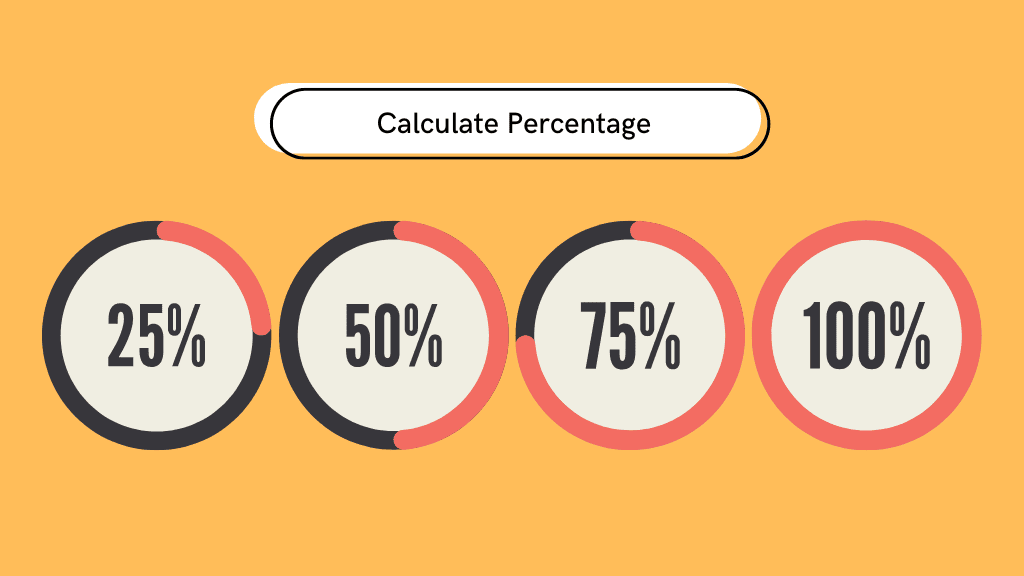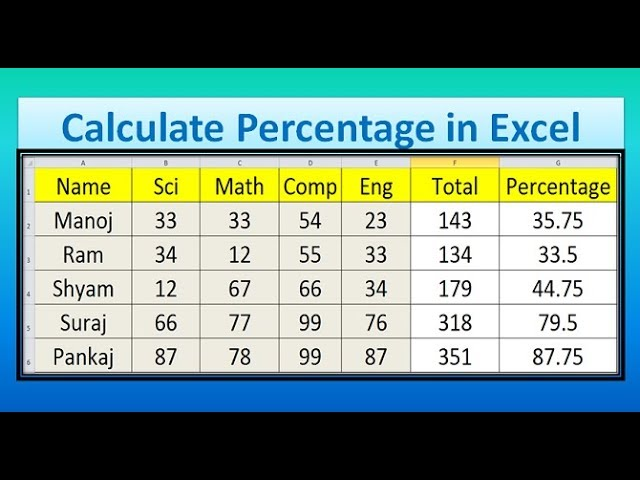# Percentage Calculation: How to Calculate Percentage of a Number, Marks and More with Formulas

Here's everything you need to know about the percentage and how you can calculate using different methods!Calculating percentages is an important mathematical skill in various real-life situations, from determining discounts at a store to analyzing statistical data. Knowing how to calculate percentages is essential not only for academic success but also for everyday decision-making. However, calculating percentages can seem daunting if you’re not comfortable with math. Fortunately, it’s not as difficult as it may seem, and with a little practice, anyone can master the art of percentage calculation.

In this article, we’ll provide a step-by-step guide on calculating percentages and some useful tips, tricks, and examples to help make the process easier. So go ahead and check out how to calculate percentages confidently and easily, no matter the situation.

## Examples of Percentage

Here are some examples of how percentages are commonly used in India:
• Percentages are utilized in many ways in India, with taxes being a prime example. The Goods and Services Tax (GST) rates fluctuate from 0% to 28%, subject to the commodity. Processed food items incur a GST rate of 5%, while luxury cars attract a 28% rate. This serves as an instance of a percentage.
• Moreover, various stores frequently offer discounts during festive seasons or sales. For instance, a saree might incur a discount of 10%, while electronics may enjoy a 20% discount.
• In addition, students’ exam results in schools and colleges are commonly expressed in percentages, such that an individual who scores 75 out of 100 on an exam would be deemed to have scored 75%.
• Furthermore, returns on diverse investment instruments such as fixed deposits, mutual funds, and stocks are typically represented in percentages. A bank fixed deposit could offer an interest rate of 7% per annum.

## How to Calculate PercentageTo calculate a percentage, there are a few simple steps that one must follow. First, determine the total value of the quantity for which the percentage is desired. Then, determine the part or portion of the total value for which the percentage is calculated. Next, divide the part or portion value by the total value. Finally, multiply the resulting value by 100 to obtain the percentage value.

To calculate a percentage, you can use the formula:

Percentage = (Part/Total) x 100

For example, let’s say you want to find out what percentage of 200 is 60, you can use the same formula:

Percentage = (60/200) x 100

Percentage = 30%

Therefore, 60 is 30% of 200.

### How to Calculate the Percentage of a NumberFor example, if you want to find out 20% of 150, you can follow these steps:

1. Write down the number 150.
2. Convert the percentage value to a decimal by dividing it by 100. So here it would be, 20% is 0.2.
3. Multiply 150 by 0.2, which is 30.

Therefore, 20% of 150 is 30.

### How to Calculate the Percentage of MarksThe formula for calculating the percentage is:

Percentage = (Obtained marks / Total marks) x 100

For example, if you scored 55 marks out of 100, then your percentage would be:

Percentage = (55 / 100) x 100

Percentage = 55%

Therefore, your percentage of marks would be 55%.

### How to Calculate Percentages in ExcelCalculating percentages in Excel is a common task that can be accomplished in a few different ways, depending on what you want to do. Here are some methods:

If you want to calculate the percentage of a number in Excel, you can follow these steps:

1. Enter the number you want to find the percentage of in a cell.
2. In another cell, enter the percentage formula: “=Number*Percentage%.”
3. Replace “Number” with the cell reference of the number you want to find the percentage of, and “Percentage” with the percentage you want to calculate (e.g., 25%).
4. The result will be the percentage of the number you entered.

If you want to calculate the percentage change between two numbers in Excel, you can use the following steps:

1. Enter the two numbers in separate cells.
2. Enter the percentage change formula in another cell: “=(New Value – Old Value)/Old Value.”
3. Replace “New Value” and “Old Value” with the cell references of the two numbers you entered.
4. The result will be the percentage change between the two numbers.

If you want to format a number as a percentage in Excel, you can use these steps:

1. Select the cells you want to format.
2. Right-click on the cell and select “Format Cells” from the drop-down menu.
3. Select “Percentage” under the “Number” tab in the Format Cells dialog box.
4. Adjust the decimal places and other formatting options as needed.

These are just a few methods that you can use to calculate percentages in Excel. Excel offers many more functions and formulas that you can use depending on your specific needs.

### How to Calculate Percentages on a CalculatorCalculating percentages on a calculator is a simple process that can be done using the following steps:

1. Enter the number you want to find the percentage of on your calculator.
2. Press the multiplication key (*).
3. Enter the percentage you want to calculate.
4. Press the percentage key (%).
5. The result will be the percentage of the number you entered.

For example, to calculate 20% of 100 on a calculator, you would follow these steps:

1. Enter 100.
2. Press the multiplication key (*).
3. Enter 20.
4. Press the percentage key (%).
5. The result will be 20, representing 20% of 100.

You can use this method to calculate any percentage of a number on your calculator, whether a simple percentage or a more complex one.